Test: Machine Instructions & Addressing Modes

# Test: Machine Instructions & Addressing Modes - Electronics and Communication Engineering (ECE)

Test Description

## 10 Questions MCQ Test Topicwise Question Bank for Electronics Engineering - Test: Machine Instructions & Addressing Modes

Test: Machine Instructions & Addressing Modes for Electronics and Communication Engineering (ECE) 2023 is part of Topicwise Question Bank for Electronics Engineering preparation. The Test: Machine Instructions & Addressing Modes questions and answers have been prepared according to the Electronics and Communication Engineering (ECE) exam syllabus.The Test: Machine Instructions & Addressing Modes MCQs are made for Electronics and Communication Engineering (ECE) 2023 Exam. Find important definitions, questions, notes, meanings, examples, exercises, MCQs and online tests for Test: Machine Instructions & Addressing Modes below.
Solutions of Test: Machine Instructions & Addressing Modes questions in English are available as part of our Topicwise Question Bank for Electronics Engineering for Electronics and Communication Engineering (ECE) & Test: Machine Instructions & Addressing Modes solutions in Hindi for Topicwise Question Bank for Electronics Engineering course. Download more important topics, notes, lectures and mock test series for Electronics and Communication Engineering (ECE) Exam by signing up for free. Attempt Test: Machine Instructions & Addressing Modes | 10 questions in 30 minutes | Mock test for Electronics and Communication Engineering (ECE) preparation | Free important questions MCQ to study Topicwise Question Bank for Electronics Engineering for Electronics and Communication Engineering (ECE) Exam | Download free PDF with solutions
 1 Crore+ students have signed up on EduRev. Have you?
Test: Machine Instructions & Addressing Modes - Question 1

### In 8051, the accumulator register contains 80H and B register contains 8FH. The content of the accumulator and status of the carry flag after the addition operation are respectively

Detailed Solution for Test: Machine Instructions & Addressing Modes - Question 1

A= 80H
B= 8FH
[A] + [B] = 80H + 8FH = 1 0FH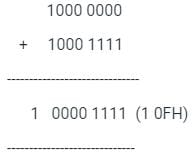But accumulator is an 8-bit register
Accumulator = 0FH
carry flag= 1

Hence the correct answer is 0FH, 1.

*Multiple options can be correct
Test: Machine Instructions & Addressing Modes - Question 2

### Which of the following statements is/are true?

Detailed Solution for Test: Machine Instructions & Addressing Modes - Question 2

Option 1: Parallelism is high in the horizontal microprogrammed control unit as compared to a vertical microprogrammed control unit.
True, Parallelism is high in horizontal microprogramming as several operations on different registers can be performed simultaneously.

Option 2: Hardwired control unit is slower compared to the microprogrammed control unit.
False, a Hardwired control unit is faster as compared to the microprogrammed control unit as there won’t be a delay of fetch, decoding, and executing the control instructions in the case of the hardwired control unit.

Option 3: In 2’s complement sum carry flag and overflow are the same.
False, In unsigned numbers, carry out is equivalent to overflow. But in two's complement, carry out tells you nothing about overflow.

Option 4: In 2’s complement sum if the sum of two negative numbers yields a positive result, the sum has overflowed.
True, the Following are the rules for detecting overflow in a two's complement sum:

• If the sum of two positive numbers yields a negative result, the sum has overflowed.
• If the sum of two negative numbers yields a positive result, the sum has overflowed.

Otherwise, the sum has not overflowed.
Hence the correct answer is option 1 and option 4.

*Answer can only contain numeric values
Test: Machine Instructions & Addressing Modes - Question 3

### Only instructions with zero, one, and two addresses are supported by some CPUs. The size of an op-code is 16 bits, whereas the size of an address is 4 bits. What is the Maximum number of two address instructions?

Detailed Solution for Test: Machine Instructions & Addressing Modes - Question 3

Commercial computers frequently have this. Two addresses can be supplied in the instruction in this case. Instead of the result being saved in the accumulator as it was in prior address instructions, the result can now be stored in many locations, however, this requires extra bits to indicate the address.

Explanation:

The given data,
The CPU supports op-code size = 16 bits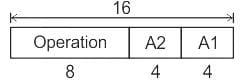We have two operands so it requires the 2 x 4 bits =8
And remaining 16-8 bits can be used for two address instructions.
i.e 8 bits.
Maximum number of instructions = 28 = 256
Hence the correct answer is 256.

Test: Machine Instructions & Addressing Modes - Question 4

Which one of the following is a special characteristic of RISC processor?

Detailed Solution for Test: Machine Instructions & Addressing Modes - Question 4

RISC means Reduced Instruction Set as the acronym says aims to reduce the execution times of instructions by simplifying the instructions.
The major characteristics of RISC are as follows:

• Compared to normal instructions they have a lower number of instructions.
• The addressing modes in the case of RISC are also lower.
• All the operations that are required to be performed take place within the CPU.
• All instructions are executed in a single cycle and hence have a faster execution time.
• The characteristic of some RISC CPUs is to use an overlapped register window that provides the passing of parameters to called procedure and stores the result to the calling procedure.
• In this architecture, the processors have a large number of registers and a much more efficient instruction pipeline.
• Also, the instruction formats are of fixed length and can be easily decoded.
Test: Machine Instructions & Addressing Modes - Question 5

A CPU has 12 registers and uses 6 addressing modes. RAM is 64K × 32. What is the maximum size of the op-code field if the instruction has a register operand and a memory address operand?

Detailed Solution for Test: Machine Instructions & Addressing Modes - Question 5

Data:
number of registers = 12
RAM size =64K × 32 = 216 × 25

Formula:
Memory Capacity is of the form = 2m × 2n
Instrcution size = Data Lines  = 2n
number of bits = ⌈log2 n⌉
number of register or number addressing modes

Calculation:
Instrcution size = Data Lines  = 32
Address lines required = 16 bits
number of bits for a addressing mode = ⌈log2 6⌉ = 3
number of bits for a register field = ⌈log2 12⌉ = 4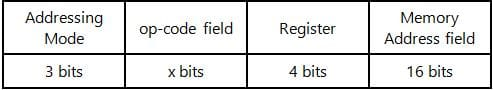3 + x + 4 + 16 = 32
∴ x = 9
op-code field = 9 bits

Test: Machine Instructions & Addressing Modes - Question 6

RISC stands for:

Detailed Solution for Test: Machine Instructions & Addressing Modes - Question 6

RISC is implemented using hardwire control unit. RISC uses registers instead of memory. Registers are small in size and are on the same chip on which ALU and control unit are present. RISC architecture is shown below.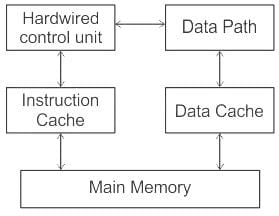Feature of RISC processor are:

• RISC instruction set are simple and of fix size.
• Fewer instructions in RISC.
• High performance
• Large number of registers.
• Instruction come under size of one word.
Test: Machine Instructions & Addressing Modes - Question 7

The following language uses mnemonic OP codes

Detailed Solution for Test: Machine Instructions & Addressing Modes - Question 7

In assembly language mnemonics are used to represent operation codes.
Opcodes (operation codes) are represented by abbreviations, called mnemonics that indicate the operation.

Mnemonic codes:

• Mnemonic codes are the codes that can be remembered comparatively easily and that aids its user in recalling the information it represents.
• Mnemonics codes are widely used in computer programming and communication system operations to specify instructions.

Examples:

• SUB        Subtract
• MUL      Multiply
• DIV         Divide
• STOR     Store data to memory
*Answer can only contain numeric values
Test: Machine Instructions & Addressing Modes - Question 8

A processor has 300 distinct instructions and 70 general-purpose registers. A 32-bit instruction word has an opcode, two register operands, and an immediate operand. The number of bits available for the immediate operand field is_____

Detailed Solution for Test: Machine Instructions & Addressing Modes - Question 8

Distinct instructions= 300
General-purpose registers = 70
opcode instruction word = 32-bit
Two register operands and an immediate operand.

Each instruction has 32 bits. To support 300 instructions, the opcode must contain 9-bits.
Register operand1 requires 7 bits, since the total registers are 70, Register operand 2 also requires 7 bits.
So, 32 - (9+7+7) = 9 bits are left over for immediate operand.

Hence the correct answer is 9.

*Multiple options can be correct
Test: Machine Instructions & Addressing Modes - Question 9

Which of the following statements is/are true?

Detailed Solution for Test: Machine Instructions & Addressing Modes - Question 9

Option 1: In the immediate addressing mode the operand is placed in the instruction itself.
True
, An immediate mode instruction has an operand field rather than an address field. The operand field contains the actual operand to be used in conjunction with the operation specified in the instruction.

Option 2: One-byte machine instruction consists of the only operand.
False, The machine instructions which consist of the only opcode are called one-byte machine instructions.

Option 3: Indirect addressing mode is suitable for implementing pointers in C.
True, Indirect addressing mode is suitable for implementing pointers in C. The instruction includes the address of the place where the target address is stored in this style of addressing. As a result, it is indirectly storing the target site's address in another memory location.

Option 4: Displacement addressing mode is similar to the register indirect addressing mode.
True,
The displacement addressing mode is equal to the register indirect addressing mode, with the exception that the effective address of the operand is formed by adding an offset (or displacement) maintained in the instruction to the contents of the register provided in the instruction.

Hence the correct answer is option 1, option 3 and option 4.

Test: Machine Instructions & Addressing Modes - Question 10

In X = (M + N × O) / (P × Q), how many one-address instructions are required to evaluate it?

Detailed Solution for Test: Machine Instructions & Addressing Modes - Question 10

All the operations will be performed in the Accumulator register(AC).
The load operation is used to fetch the value from register or memory to accumulator.
The store operation is used to store the value from the accumulator to register or memory.
The one address instructions for the given equations are:​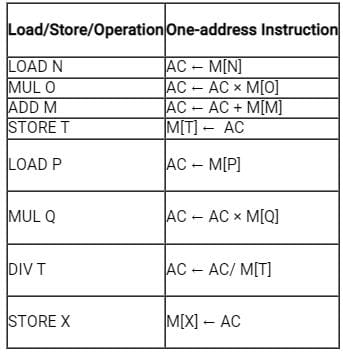Hence, the correct answer is "option 3".

## Topicwise Question Bank for Electronics Engineering

193 tests(Scan QR code)